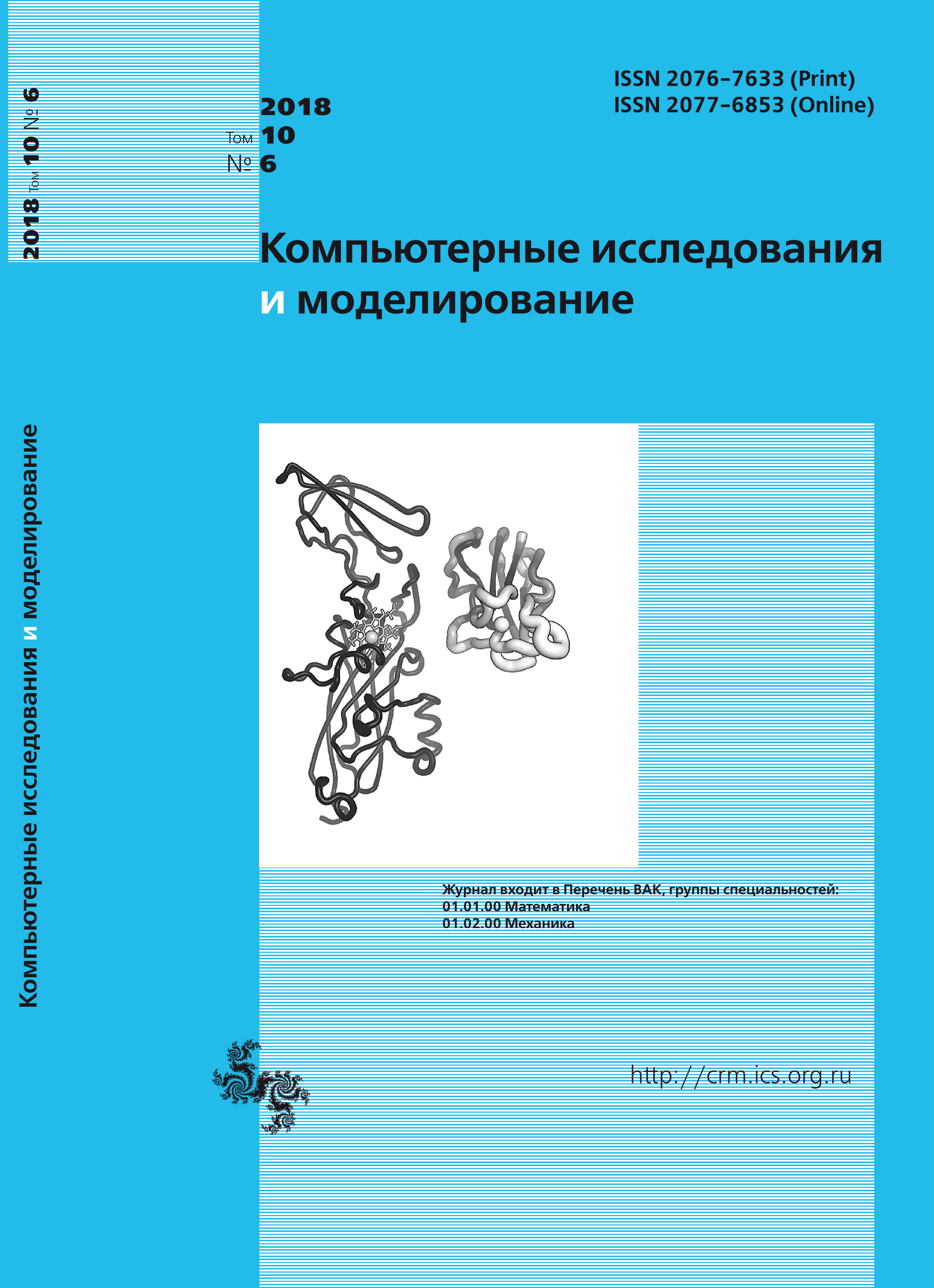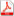Issue 6, 2018 Vol. 10

# All issues

Estimation of models parameters for time series with Markov switching regimespdf (2286K)  / List of references

The paper considers the problem of estimating the parameters of time series described by regression models with Markov switching of two regimes at random instants of time with independent Gaussian noise. For the solution, we propose a variant of the EM algorithm based on the iterative procedure, during which an estimation of the regression parameters is performed for a given sequence of regime switching and an evaluation of the switching sequence for the given parameters of the regression models. In contrast to the well-known methods of estimating regression parameters in the models with Markov switching, which are based on the calculation of a posteriori probabilities of discrete states of the switching sequence, in the paper the estimates are calculated of the switching sequence, which are optimal by the criterion of the maximum of a posteriori probability. As a result, the proposed algorithm turns out to be simpler and requires less calculations. Computer modeling allows to reveal the factors influencing accuracy of estimation. Such factors include the number of observations, the number of unknown regression parameters, the degree of their difference in different modes of operation, and the signal-to-noise ratio which is associated with the coefficient of determination in regression models. The proposed algorithm is applied to the problem of estimating parameters in regression models for the rate of daily return of the RTS index, depending on the returns of the S&P 500 index and Gazprom shares for the period from 2013 to 2018. Comparison of the estimates of the parameters found using the proposed algorithm is carried out with the estimates that are formed using the EViews econometric package and with estimates of the ordinary least squares method without taking into account regimes switching. The account of regimes switching allows to receive more exact representation about structure of a statistical dependence of investigated variables. In switching models, the increase in the signal-to-noise ratio leads to the fact that the differences in the estimates produced by the proposed algorithm and using the EViews program are reduced.

Keywords: estimation of parameters, regression models, Markov switching models, likelihood function, maximum likelihood method, noise variance, signal to noise ratio
Citation in English: Silaeva V.A., Silaeva M.V., Silaev A.M. Estimation of models parameters for time series with Markov switching regimes // Computer Research and Modeling, 2018, vol. 10, no. 6, pp. 903-918
Citation in English: Silaeva V.A., Silaeva M.V., Silaev A.M. Estimation of models parameters for time series with Markov switching regimes // Computer Research and Modeling, 2018, vol. 10, no. 6, pp. 903-918
DOI: 10.20537/2076-7633-2018-10-6-903-918
Views (last year): 36.

Full-text version of the journal is also available on the web site of the scientific electronic library eLIBRARY.RU

The journal is included in the Russian Science Citation Index

The journal is included in the List of Russian peer-reviewed journals publishing the main research results of PhD and doctoral dissertations.

International Interdisciplinary Conference "Mathematics. Computing. Education"

The journal is included in the RSCI

Indexed in Scopus